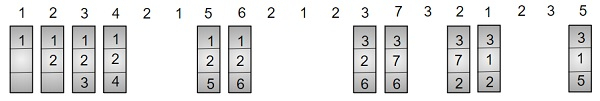# C++ Program for Optimal Page Replacement Algorithm

Given page number and page size; the task is to find number of hits and misses as when we allocate the memory block to a page using Optimal Page Replacement Algorithm.

What is Optimal Page Replacement Algorithm?

Optimal page replacement algorithm is a page replacement algorithm. A page replacement algorithm is an algorithm which decides which memory page is to be replaced. In Optimal page replacement we replace the page which is not referred to the near future, although it can’t be practically implemented, but this is most optimal and have minimal miss, and is most optimal.

Let’s understand by using an example and explaining it diagrammatically.Here after allocating 1, 2 and 3 now the memory is full, so for inserting 4 we will look for the page which is not again referred in near future from 1, 2 and 3 so page 3 is not in near future so we replace that page with new page 4, and so on we will repeat the steps till we reach the end.

## Example

Input: page[] = { 1, 7, 8, 3, 0, 2, 0, 3, 5, 4, 0, 6, 1 }
fn=3
Output: Hits = 3
Misses = 10

Input: page[] = { 7, 0, 1, 2, 0, 3, 0, 4, 2, 3, 0, 3, 2 }
fn = 4
Output: Hits = 7
Misses= 6

Approach we are using to solve the above problem −

• Take the input of pages as an array.
• Look for the page allocated is present in near future, if no then replace that page in the memory with new page,
• If page already present increment hit, else increment miss.
• Repeat till we reach the last element of the array.
• Print the number of hits and misses.

## Algorithm

Start
Step 1-> In function int predict(int page[], vector<int>& fr, int pn, int index)
Declare and initialize res = -1, farthest = index
Loop For i = 0 and  i < fr.size() and i++
Loop For j = index and j < pn and j++
If fr[i] == page[j] then,
If j > farthest
Set farthest = j
End If
Set res = i
break
If j == pn then,
Return i
Return (res == -1) ? 0 : res
Step 2-> In function bool search(int key, vector<int>& fr)
Loop For i = 0 and i < fr.size() and i++
If fr[i] == key then,
Return true
Return false
Step 3-> In function void opr(int page[], int pn, int fn)
Declare vector<int> fr
Set hit = 0
Loop For i = 0 and i < pn and i++
If search(page[i], fr) then,
Increment hit by 1
continue
If fr.size() < fn then,
fr.push_back(page[i])
Else
Set j = predict(page, fr, pn, i + 1)
Set fr[j] = page[i]
Print the number of hits
Print the number of misses
Step 4-> In function int main()
Declare and assign page[] = { 1, 7, 8, 3, 0, 2, 0, 3, 5, 4, 0, 6, 1 }
Set pn = sizeof(page) / sizeof(page)
Set fn = 3
opr(page, pn, fn)
Stop

## Example

Live Demo

#include <bits/stdc++.h>
using namespace std;
int predict(int page[], vector<int>& fr, int pn, int index) {
// Store the index of pages which are going
// to be used recently in future
int res = -1, farthest = index;
for (int i = 0; i < fr.size(); i++) {
int j;
for (j = index; j < pn; j++) {
if (fr[i] == page[j]) {
if (j > farthest) {
farthest = j;
res = i;
}
break;
}
}
// Return the page which are
// are never referenced in future,
if (j == pn)
return i;
}
// If all of the frames were not in future,
// return any of them, we return 0. Otherwise
// we return res.
return (res == -1) ? 0 : res;
}
bool search(int key, vector<int>& fr) {
for (int i = 0; i < fr.size(); i++)
if (fr[i] == key)
return true;
return false;
}
void opr(int page[], int pn, int fn) {
vector<int> fr;
int hit = 0;
for (int i = 0; i < pn; i++) {
// Page found in a frame : HIT
if (search(page[i], fr)) {
hit++;
continue;
}
// check if there is space available in frames.
if (fr.size() < fn)
fr.push_back(page[i]);
// Find the page to be replaced.
else {
int j = predict(page, fr, pn, i + 1);
fr[j] = page[i];
}
}
cout << "Hits = " << hit << endl;
cout << "Misses = " << pn - hit << endl;
}
// main Function
int main() {
int page[] = { 1, 7, 8, 3, 0, 2, 0, 3, 5, 4, 0, 6, 1 };
int pn = sizeof(page) / sizeof(page);
int fn = 3;
opr(page, pn, fn);
return 0;
}

## Output

Hits = 3
Misses = 10